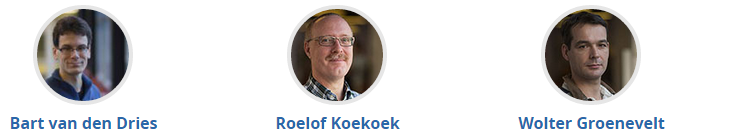## TOPICS, RELEASE DATES & RECOMMENDED DEADLINES

WeekTopic
1

Functions (part 1)

In week 1 you will be introduced to mathematical modeling and the role of functions to model real-world phenomena. You will learn what a function is and how to describe a function in text, graph, table and formula. You will learn the characteristics of polynomials, rational functions and power functions and their rules of calculation.

2

Functions (part 2)

In week 2 you will familiarize yourself with other elementary functions: trigonometric functions (sine, cosine and tangent) and exponential functions and their inverse: logarithms.

3

Equations (part 1)

In week 3 you will learn the role of equations in mathematical modeling. You will learn to solve equations involving polynomials, fractions and square roots. You will also learn general techniques to solve equations.

4

Equations (part 2)

In week 4 you will solve equations involving trigonometric functions (sine, cosine and tangent) and exponential functions and logarithms. You will also learn to solve inequalities and systems of equations.

5

Differentiation

In week 5 you will be introduced to differentiation using speed and slope as an example. You will learn to calculate the derivative and practice with the rules of calculation. You will see applications of differentiation, especially to finding maxima and minima of functions.

6

Integration

In week 6 you will be introduced to integration. You will learn the definition and the fundamental theorem of Calculus that relates differentiation and integration. You will also practice with the rules of calculation for integrals.

7

Exam

The course finishes with a final exam.

The course has only 1 deadline: September 29, 2016, 23.30 hours UTC (Universal Time Coordinated). At that moment the final scores for the exercises, homework and final exam are counted and the certificates are issued to those with sufficient scores. However we recommend to make your own planning and set intermediate deadlines well in advance of the final deadline.

## main Learning objectives

By the end of the course, you will be able to:

• Manipulate elementary functions, like power functions, rational functions, polynomials, trigonometric functions, exponential and logarithmic functions. (Weeks 1 and 2)
• Solve equations and inequalities involving these elementary functions. (Weeks 3 and 4)
• Use the concept of differentiation and calculate the derivatives of compositions of elementary functions. (Week 5)
• Use the concept of integration and use some elementary integration techniques. (Week 6)

## Grading & Certification

This course contains three types of activities that contribute to the final grade:

1. Exercises (12%)
2. Homework (60%)
3. Final exam (28%)

The exercises are exercises that are given after videos to practice immediately with the content. The homework problems are positioned at the end of each week. The final exam is in week 7 and contains problems on all the topics. Every week also contains a training sequence. Those training questions are NOT graded.

In the table below you see how many percent of the total score  you can earn each week with the exercises and the homework and which percentage you can obtain with the final exam.

 Week 1 Week 2 Week 3 Week 4 Week 5 Week 6 Week 7 Total Exercises 2% 2% 2% 2% 2% 2% 12% Training 0% 0% 0% 0% 0% 0% Homework 10% 10% 10% 10% 10% 10% 60% Final exam 28% 28% 100%

Your score will be calculated by multiplying your percentage of correct answers per item (for example exercises week 1: 90% correct) by the weight percentage in the table above (in the case of the example: 2%) and then adding all the items.

The verified certificate is issued if your total score is 60% or more. Your final grade will be your calculated score percentage. The verified certificate will be issued on the week after the course ends.

You can check your grade at any time under the course’s Progress Tab.

Remember, you have until September 29, 2016, 23.30 hours UCT to finish all the modules and tests.

Please note that this MOOC is not part of a formal deficiency program or will lead to exemptions of certain deficiencies if you want to enroll in a bachelor program at the TU Delft.

The estimated workload for this course is 6 to 8 hours per week, so about 50 hours in total.

## teachers## Prerequisites

The aim of this course is to review important topics in high school level mathematics. As such, we assume high school mathematics as prerequisite.